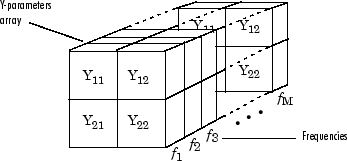Y-Parameters Passive Network

Model passive network using Y-parameters

• Library:
• RF Blockset / Equivalent Baseband / Black Box Elements

•Description

The Y-Parameters Passive Network block models the two-port passive network described in the block dialog box, in terms of its Y-parameters and their associated frequencies.

In the Y-Parameters field of the block dialog box, provide the Y-parameters for each of the M frequencies as a 2-by-2-by-M array. In the Frequency field, specify the frequencies for the Y-parameters as an M-element vector. The elements of the vector must be in the same order as the Y-parameters. The figure shows the correspondence between the Y-parameters array and the vector of frequencies.The Y-Parameters Passive Network block uses the RF Toolbox™ y2s function to convert the Y-parameters to S-parameters, and then interpolates the resulting S-parameters to determine their values at the modeling frequencies. The modeling frequencies are determined by the Output Port block. See Map Network Parameters to Modeling Frequencies for more details.

Parameters

expand all

Main

Y-parameters for a two-port passive network, specified as a 2-by-2-by-M array where M is the number of Y-parameters.

Frequency of Y-parameters, specified as an M-element vector with each element unit in hertz. All frequencies must be positive.

Method to interpolate the network parameters, specified as one of the following:

MethodDescription
LinearLinear interpolation
SplineCubic spline interpolation
CubicPiecewise cubic Hermite interpolation

Visualization

Frequency data source, specified as Same as the Y-Parameters or User-specified.

Frequency data range, specified as a vector with each element unit in hertz.

Type of data plot to visualize using the given data, specified as one of the following:

• X-Y plane — Generate a Cartesian plot of the data versus frequency. To create linear, semilog, or log-log plots, set the Y-axis scale and X-axis scale accordingly.

• Composite data — Plot the composite data. For more information, see Create Plots.

• Polar plane — Generate a polar plot of the data. The block plots only the range of data corresponding to the specified frequencies.

• Z smith chart, Y smith chart, and ZY smith chart — Generate a Smith® chart. The block plots only the range of data corresponding to the specified frequencies.

Type of parameters to plot, specified as one of the following:

 S11 S12 S21 S22 GroupDelay GammaIn GammaOut VSWRIn VSWROut OIP3 IIP3 NF NFactor NTemp TF1 TF2 TF3 Gt Ga Gp Gmag Gmsg GammaMS GammaML K Delta Mu MuPrime

Note

Y parameter1 is disabled when you select Plot type to Composite data.

Type of parameters to plot, specified as one of the following:

 S11 S12 S21 S22 GroupDelay GammaIn GammaOut VSWRIn VSWROut OIP3 IIP3 NF NFactor NTemp TF1 TF2 TF3 Gt Ga Gp Gmag Gmsg GammaMS GammaML K Delta Mu MuPrime

Note

Y parameter2 is disabled when you select Plot type to Composite data.

Plot format, specified as one of the following.

Y parameter1Y format1
S11, S12, S21, S22, GammaIn, GammaOut, TF1, TF2, TF3, GammaMS, GammaML, and Delta.dB, Magnitude (decibels), Abs, Mag, Magnitude (linear), Angle, Angle(degrees), Angle(radians), Real, Imag, and Imaginary.
GroupDelayns, us, ms, s, and ps.
VSWRIn and VSWROut.Magnitude (decibels) and None.
OIP3 and IIP3.dBm, W, and mW.
NF dB and Magnitude (decibels).
NFactor, K, Mu, and MuPrime.None
NTempKelvin
Gt, Ga, Gp, Gmag, and Gmsg.dB, Magnitude (decibels), and None.

Dependencies

To enable Y format1, set Plot type to X-Y plane.

Plot format, specified as one of the following.

Y parameter2Y format2
S11, S12, S21, S22, GammaIn, GammaOut, TF1, TF2, TF3, GammaMS, GammaML, and Delta.dB, Magnitude (decibels), Abs, Mag, Magnitude (linear), Angle, Angle(degrees), Angle(radians), Real, Imag, and Imaginary.
GroupDelayns, us, ms, s, and ps.
VSWRIn and VSWROut.Magnitude (decibels) and None.
OIP3 and IIP3.dBm, W, and mW.
NF dB and Magnitude (decibels).
NFactor, K, Mu, and MuPrime.None
NTempKelvin
Gt, Ga, Gp, Gmag, and Gmsg.dB, Magnitude (decibels), and None.

Dependencies

To enable Y format2, set Plot type to X-Y plane.

Frequency plot, specified as Freq.

Frequency plot format, specified as one of the following.

 Auto Hz kHz MHz GHz THz

Y-axis scale, specified as Linear or Log.

Dependencies

To enable this parameter, set Plot type to X-Y plane.

X-axis scale, specified as Linear or Log.

Dependencies

To enable this parameter, set Plot type to X-Y plane.

Plot specified data using the plot button.

Introduced before R2006a